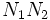# Congruence condition on number of elementary abelian subgroups of prime-square order for odd prime

This article states and (possibly) proves a fact that is true for odd-order p-groups: groups of prime power order where the underlying prime is odd. The statement is false, in general, for groups whose order is a power of two.
View other such facts for p-groups|View other such facts for finite groups
View a complete list of replacement theorems| View a complete list of failures of replacement
View other congruence conditions

## Statement

### Hands-on statement

Suppose$p$ is an odd prime and$G$ is a finite$p$-group. Suppose$G$ has an elementary abelian subgroup of order$p^2$. Then, the following are true:

1. The number of elementary abelian subgroups of$G$ of order$p^2$ is congruent to$1$ modulo$p$.
2. The number of elementary abelian normal subgroups of$G$ of order$p^2$ is congruent to$1$ modulo$p$. In particular, there is an elementary abelian normal subgroup of order$p^2$.
3. If$G$ is a normal subgroup of a bigger finite$p$-group$L$ and$G$ contains an elementary abelian subgroup of order$p^2$, then the number of elementary abelian subgroups of$G$ that are normal in$L$ is congruent to$1$ modulo$p$. In particular, there is an elementary abelian subgroup of$G$ that is normal in$L$.

### Statement in terms of a universal congruence condition

Let$p$ be an odd prime.

Let$\mathcal{S}$ be a singleton set comprising the elementary abelian subgroup of order$p^2$.

Then,$\mathcal{S}$ is a Collection of groups satisfying a universal congruence condition (?) for the prime$p$.

## Proof

We prove here the stronger version.

### Equivalence of conditions (1)-(3)

For the equivalence of (1) and (2), consider the action of$G$ on itself by conjugation. Under this action, the non-normal elementary abelian subgroups of order$p^2$ form orbits whose size is a multiple of$p$. Thus, the number of elementary abelian subgroups of order$p^2$ is congruent modulo$p$ to the number of elementary abelian normal subgroups of order$p^2$.

For the equivalence of definitions (1) and (3), consider the action of$L$ on$G$ by conjugation. The subgroups of$G$ that are not normal in$L$ have orbits whose sizes are multiples of$p$, so the number of elementary abelian subgroups of order$p^2$ in$G$ is congruent modulo$p$ to the number of such subgroups that are normal in$L$. In particular, (1) implies (3). (3) clearly implies (2), and (2) is equivalent to (1).

We will freely use this equivalence in the proof below.

### Main proof

Given: A$p$-group$G$ (odd$p$).$G$ has an elementary abelian subgroup$K$ of order$p^2$.

To prove: The number of elementary abelian subgroups of$G$ of order$p^2$ is$1$ modulo$p$.

Proof: We prove the claim by induction on$G$, assuming the result is true for smaller orders.

If$K = G$, the number of subgroups is$1$, and we are done. We consider the other case:

1. There exists a maximal subgroup$M$ of$G$ containing$K$: This follows since$K$ is proper.
2.$M$ is normal in$G$: This follows from fact (1).
3.$G$ contains an elementary abelian normal subgroup$N$ of order$p^2$ (in fact,$N \le M$): We apply the induction hypothesis in form (3) with$M$ in place of$G$ and$G$ in place of$L$.
4. If$G$ contains only one elementary abelian normal subgroup of order$p^2$, we've proved the statement for$G$ in form (2). So, we assume that$G$ contains two distinct elementary abelian normal subgroups$N_1,N_2$.
5. Consider the product$N_1N_2$. This is either elementary abelian of order$p^4$, or is isomorphic to prime-cube order group:U(3,p), i.e., is a non-abelian group of order$p^3$ and exponent$p$. In either case, every maximal subgroup of it contains an elementary abelian subgroup of order$p^2$ (for more details, see fact (3)). In particular, the intersection of$N_1N_2$ with any maximal subgroup of$G$ contains an elementary abelian group of order$p^2$.
6. Thus,$N_1N_2$ is a local origin in the terminology of fact (2), and the induction hypothesis yields that the number of elementary abelian subgroups of order$p^2$ in$G$ is congruent to$1$ modulo$p$.